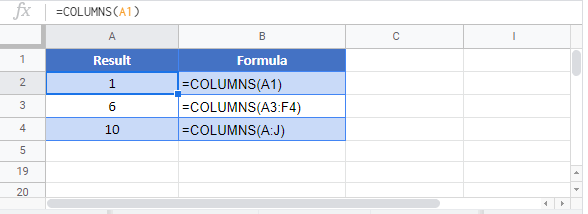# COLUMNS Function Examples – Excel, VBA, & Google Sheets

This Tutorial demonstrates how to use the Excel COLUMNS Function in Excel to count the number of columns.

## COLUMNS Function Overview

The COLUMNS Function Calculates the number of columns in an array.

To use the COLUMNS Excel Worksheet Function, select a cell and type: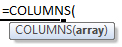(Notice how the formula inputs appear)

## COLUMNS function Syntax and inputs:

array – Count the number of columns in this array.

## COLUMNS Function

The COLUMNS Function returns the number of columns in a given cell reference.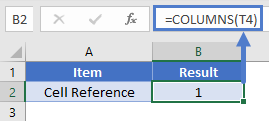## COLUMNS Function – Cell Range

You can also enter a cell range and it will return the total number of columns in it.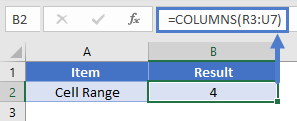## COLUMNS Function – Array Constant

You can also count the number of columns in a given array constant.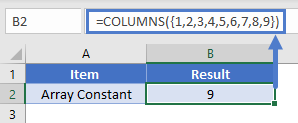## COLUMNS Function in Google Sheets

The COLUMNS Function works exactly the same in Google Sheets as in Excel: